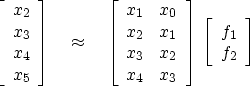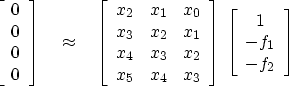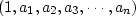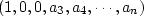Next: Prediction-error filters on synthetic Up: Time-series analysis Previous: Random numbers into the

# THE ERROR FILTER FAMILY

A simple regression for a prediction filter (f1,f2) is(9)
Notice that each row in this equation says that xt fits a linear combination of x at earlier times; hence the description of f as a prediction" filter. The error in the prediction is simply the left side minus the right side. Rearranging the terms, we get(10)
We have already written programs for regressions like (9). Regressions like (10), however, often arise directly in practice. They are easier to solve directly than by transforming them to resemble (9).

Multiple reflections are predictable. It is the unpredictable part of a signal, the prediction residual, that contains the primary information. The output of the filter (1,-f1, -f2) is the unpredictable part of the input. This filter is a simple example of a prediction-error" (PE) filter. It is one member of a family of filters called error filters."

The error-filter family are filters with one coefficient constrained to be unity and various other coefficients constrained to be zero. Otherwise, the filter coefficients are chosen to have minimum power output. Names for various error filters follow:prediction-error (PE) filtergapped PE filter with a gap of 2interpolation-error (IE) filtera gapped IE filter


A program for computing all the error filters will be presented after we examine a collection of examples. 1Next: Prediction-error filters on synthetic Up: Time-series analysis Previous: Random numbers into the
Stanford Exploration Project
10/21/1998